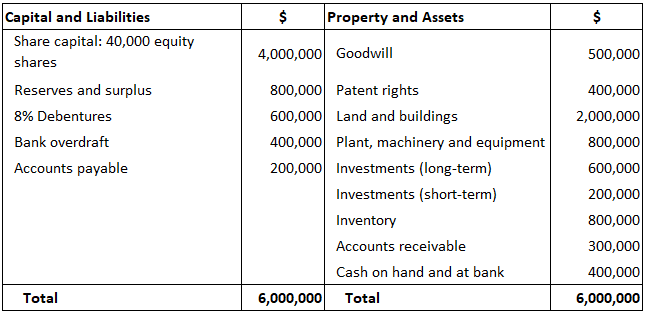## What Is Carrying Value?

Carrying value or book value is the value of an asset according to the figures shown (carried) in a company’s balance sheet. Carrying value is calculated as the original cost of the asset less any depreciation, amortization, or impairment costs.

### Formula to Calculate Carrying or Book Value

Book Value or Carrying Value = Total Assets – Total Liabilities
Tangible Book Value = Tangible Assets – Total Liabilities
In the second formula, tangible assets is equal to (total assets – goodwill and intangible assets).

### Uses of Carrying or Book Value

Carrying value has two main uses:

1. When carrying value is compared to an enterprise’s market value, it can indicate whether a stock is underpriced or overpriced.
2. In personal finance, an investment’s carrying value is the price paid for it in shares/stock or debt. When this stock or debt is sold, the selling price less the book value is the capital gain/loss from investment.

Therefore, carrying value is the accounting value of the enterprise. In other words, it is the total value of the enterprise’s assets that owners (shareholders) would theoretically receive if an enterprise was liquidated.

In reality, carrying value does not always reflect what shareholders will receive in the event of liquidation. For example, even if the inventory is stated at full cost (100% value) in the books, who would want to buy a bunch of Pentium IV chips if the company goes out of business?

This means that the realization value of assets of ongoing concern is different from the value of assets under liquidation.

It is important to predict the fair value of all assets when an enterprise stops its operations. Generally, it is estimated that the fair values of cash and cash equivalents, short-term investments (less than one year), and long-term investments (beyond one year) are equal to 100% of the book value.

The fair values of different items are given below:

• Accounts receivable (Debtors + B/R) and inventory items are equal to 50% of the carrying value.
• Plant and equipment items are equal to around 25% of the carrying value.
• Land and buildings, in a business-oriented city, may be beyond 100% of the carrying value.
• Goodwill and intangible items are equal to 0%.
• Liabilities, because they should be paid in full, have a fair value equal to 100% of the carrying value.

Hence, if an enterprise undergoes liquidation, the fair value prediction of assets clearly indicates that the owners (shareholders) cannot receive the net carrying value of assets.

Importantly, this thought process for determining carrying value versus fair value prediction paves the way for the concept of entity value (or enterprise value), which is a holistic measure of organizational value.

## Example

Using the table below, calculate the following:

1. Carrying value of the assets
2. Tangible book value of the assets### Solution

1. Carrying Value = Total Assets – Total Liabilities
\$6,000,000 – \$1,200,000 = \$4,800,000
Liabilities = Debentures + Bank Overdraft + Accounts Payable
= \$600,000 + \$400,000 + \$200,000 = \$1,200,000
2. Tangible Book Value = Total Tangible Assets – Total Liabilities
= \$5,100,000 – \$1,200,000 = \$3,900,000
Tangible Assets = Total Assets – (Goodwill + Patent Rights)
= \$6,000,000 – (\$500,000 + \$400,000) = \$5,100,000

### What is the carrying value?

The carrying value of an asset is its net worth—the amount at which the asset is currently valued on the balance sheet.

### How do I calculate the carrying values?

The carrying values of an asset can be calculated by subtracting the total liabilities of that particular asset from its total assets. In case the value obtained is negative, it means that the asset has a net loss or it can be said that its losses exceed its profits, thus making it a liability.

### What are the uses of a carrying value?

In personal finance, an investment’s carrying value is the price paid for it in shares/stock or debt. When this stock or debt is sold, the selling price less the book value is the capital gain/loss from an investment. Therefore, carrying value is the accounting value of the enterprise. In other words, it is the total value of the enterprise's assets that owners would theoretically receive if an enterprise was liquidated.

### What is the difference between a carrying value and a book value?

Book value is the accounting value of an asset. Carrying value, on the other hand, is the theoretical or expected exit (liquidation) value that shareholders would receive if they were to sell all their assets and pay off all associated debt at once. So, your book values are actual values that you list in your financial reports while your carrying values are the 'estimated' or 'appraised value' that you would get if you were to sell off all your assets.

### What is the difference between a book value and a fair market value?

All three terms can be used interchangeably because they refer to the same thing - the true market value of an asset at any given point in time.

True is a Certified Educator in Personal Finance (CEPF®), a member of the Society for Advancing Business Editing and Writing, contributes to his financial education site, Finance Strategists, and has spoken to various financial communities such as the CFA Institute, as well as university students like his Alma mater, Biola University, where he received a bachelor of science in business and data analytics.

To learn more about True, visit his personal website, view his author profile on Amazon, his interview on CBS, or check out his speaker profile on the CFA Institute website.